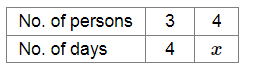# Tick (✓) the correct answer

Question:

3 persons can build a wall in 4 days, then 4 persons can build it in

(a) $5 \frac{1}{3}$ days

(b) 3 days

(c) $4 \frac{1}{3}$ days

(d) none of these

Solution:

(b) 3 days

Let x be number of days taken by 4 persons to build the wall.More number of persons will take less time to build the wall.

So, it is a case of inverse proportion.

Now, $3 \times 4=4 \times x$

$\Rightarrow x=3$

Therefore, 4 persons can build the wall in 3 days.